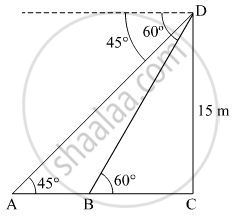Share

# Two Points a and B Are on the Same Side of a Tower and in the Same Straight Line with Its Base. the Angles of Depression of These Points from the Top of the Tower Are 60° and 45° Respectively. If the Height of the Tower is 15 M, Then Find the Distance Between the Points. - CBSE Class 10 - Mathematics

#### Question

Two points A and B are on the same side of a tower and in the same straight line with its base. The angles of depression of these points from the top of the tower are 60° and 45° respectively. If the height of the tower is 15 m, then find the distance between the points.

#### Solution

Let CD be the tower. A and B are the two points on the same side of the tower.In ΔDBC

tan 60^@ = (DC)/(BC)

=> sqrt3 = 15/(BC)

=> BC = 15/sqrt3

=> BC = 5sqrt3 m

In ΔDAC

tan 45^@ = (DC)/(AC)

=> 1 = 15/"AC"

=> AC = 15 m

Now

AC = AB + BC

:. AB = AC - BC = 15 - 5sqrt3 = 5(3 - sqrt3) m

Hence, the distance between the two points A and B is 5(3 - sqrt3) m

Is there an error in this question or solution?

#### Video TutorialsVIEW ALL 

Solution Two Points a and B Are on the Same Side of a Tower and in the Same Straight Line with Its Base. the Angles of Depression of These Points from the Top of the Tower Are 60° and 45° Respectively. If the Height of the Tower is 15 M, Then Find the Distance Between the Points. Concept: Heights and Distances.
S